### Example 41.14 Generalized Poisson Mixed Model for Overdispersed Count Data

Overdispersion is the condition by which data appear more dispersed than is expected under a reference model. For count data, the reference models are typically based on the binomial or Poisson distributions. Among the many reasons for overdispersion are an incorrect model, an incorrect distributional specification, incorrect variance functions, positive correlation among the observations, and so forth. In short, correcting an overdispersion problem, if it exists, requires the appropriate remedy. Adding an R-side scale parameter to multiply the variance function is not necessarily the adequate correction. For example, Poisson-distributed data appear overdispersed relative to a Poisson model with regressors when an important regressor is omitted.

If the reference model for count data is Poisson, a number of alternative model formulations are available to increase the dispersion. For example, zero-inflated models add a proportion of zeros (usually from a Bernoulli process) to the zeros of a Poisson process. Hurdle models are two-part models where zeros and nonzeros are generated by different stochastic processes. Zero-inflated and hurdle models are described in detail by Cameron and Trivedi (1998) and cannot be fit with the GLIMMIX procedure. See Section 15.5 in Littell et al. (2006) for examples of using the NLMIXED procedure to fit zero-inflated and hurdle models.

An alternative approach is to derive from the reference distribution a probability distribution that exhibits increased dispersion. By mixing a Poisson process with a gamma distribution for the Poisson parameter, for example, the negative binomial distribution results, which is thus overdispersed relative to the Poisson.

Joe and Zhu (2005) show that the generalized Poisson distribution can also be motivated as a Poisson mixture and hence provides an alternative to the negative binomial (NB) distribution. Like the NB, the generalized Poisson distribution has a scale parameter. It is heavier in the tails than the NB distribution and easily reduces to the standard Poisson. Joe and Zhu (2005) discuss further comparisons between these distributions.

The probability mass function of the generalized Poisson is given by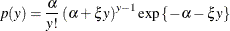where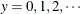,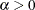, and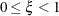(Joe and Zhu, 2005). Notice that for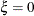the mass function of the standard Poisson distribution with meanresults. The mean and variance of Y in terms of the parametersand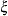are given by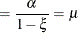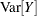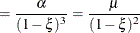The log likelihood of the generalized Poisson can thus be written in terms of the meanand scale parameteras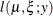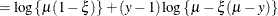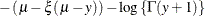The data in the following DATA step are simulated counts. For each of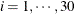subjects a randomly varying number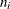of observations were drawn from a count regression model with a single covariate and excess zeros (compared to a Poisson distribution).

data counts;
input ni @@;
sub = _n_;
do i=1 to ni;
input x y @@;
output;
end;
datalines;
1 29 0
6  2 0 82 5 33 0 15 2 35 0 79 0
19 81 0 18 0 85 0 99 0 20 0 26 2 29 0 91 2 37 0 39 0  9 1 33 0
3 0 60 0 87 2 80 0 75 0  3 0 63 1
9 18 0 64 0 80 0  0 0 58 0  7 0 81 0 22 3 50 0
15 91 0  2 1 14 0  5 2 27 1  8 1 95 0 76 0 62 0 26 2  9 0 72 1
98 0 94 0 23 1
2 34 0 95 0
18 48 1  5 0 47 0 44 0 27 0 88 0 27 0 68 0 84 0 86 0 44 0 90 0
63 0 27 0 47 0 25 0 72 0 62 1
13 28 1 31 0 63 0 14 0 74 0 44 0 75 0 65 0 74 1 84 0 57 0 29 0
41 0
9 42 0  8 0 91 0 20 0 23 0 22 0 96 0 83 0 56 0
3 64 0 64 1 15 0
4  5 0 73 2 50 1 13 0
2  0 0 41 0
20 21 0 58 0  5 0 61 1 28 0 71 0 75 1 94 16 51 4 51 2 74 0  1 1
34 0  7 0 11 0 60 3 31 0 75 0 62 0 54 1
2 66 1 13 0
5 83 7 98 1 11 1 28 0 18 0
17 29 5 79 0 39 2 47 2 80 1 19 0 37 0 78 1 26 0 72 1  6 0 50 3
50 4 97 0 37 2 51 0 45 0
17 47 0 57 0 33 0 47 0  2 0 83 0 74 0 93 0 36 0 53 0 26 0 86 0
6 0 17 0 30 0 70 1 99 0
7 91 0 25 1 51 4 20 0 61 1 34 0 33 2
14 60 0 87 0 94 0 29 0 41 0 78 0 50 0 37 0 15 0 39 0 22 0 82 0
93 0  3 0
16 68 0 26 1 19 0 60 1 93 3 65 0 16 0 79 0 14 0  3 1 90 0 28 3
82 0 34 0 30 0 81 0
19 48 3 48 1 43 2 54 0 45 9 53 0 14 0 92 5 21 1 20 0 73 0 99 0
66 0 86 2 63 0 10 0 92 14 44 1 74 0
8 34 1 44 0 62 0 21 0  7 0 17 0  0 2 49 0
13 11 0 27 2 16 1 12 3 52 1 55 0  2 6 89 5 31 5 28 3 51 5 54 13
64 0
9  3 0 36 0 57 0 77 0 41 0 39 0 55 0 57 0 88 1
7  2 0 80 0 41 1 20 0  2 0 27 0 40 0
18 73 1 66 0 10 0 42 0 22 0 59 9 68 0 34 1 96 0 30 0 13 0 35 0
51 2 47 0 60 1 55 4 83 3 38 0
17 96 0 40 0 34 0 59 0 12 1 47 0 93 0 50 0 39 0 97 0 19 0 54 0
11 0 29 0 70 2 87 0 47 0
13 59 0 96 0 47 1 64 0 18 0 30 0 37 0 36 1 69 0 78 1 47 1 86 0
88 0
15 66 0 45 1 96 1 17 0 91 0  4 0 22 0  5 2 47 0 38 0 80 0  7 1
38 1 33 0 52 0
12 84 6 60 1 33 1 92 0 38 0  6 0 43 3 13 2 18 0 51 0 50 4 68 0
;


The following PROC GLIMMIX statements fit a standard Poisson regression model with random intercepts by maximum likelihood. The marginal likelihood of the data is approximated by adaptive quadrature (METHOD=QUAD).

proc glimmix data=counts method=quad;
class sub;
model y = x / link=log s dist=poisson;
random int / subject=sub;
run;


Output 41.14.1 displays various informational items about the model and the estimation process.

Output 41.14.1: Poisson: Model and Optimization Information

The GLIMMIX Procedure

Model Information
Data Set WORK.COUNTS
Response Variable y
Response Distribution Poisson
Variance Function Default
Variance Matrix Blocked By sub
Estimation Technique Maximum Likelihood
Degrees of Freedom Method Containment

Optimization Information
Optimization Technique Dual Quasi-Newton
Parameters in Optimization 3
Lower Boundaries 1
Upper Boundaries 0
Fixed Effects Not Profiled
Starting From GLM estimates

Iteration History
Iteration Restarts Evaluations Objective
Function
Change Max
0 0 4 862.57645728 . 366.7105
1 0 5 862.43893582 0.13752147 22.36158
2 0 6 854.49131023 7.94762559 28.70814
3 0 2 854.47983504 0.01147519 6.036114
4 0 4 854.47396189 0.00587315 4.238363
5 0 4 854.47006558 0.00389631 0.332454
6 0 3 854.47006484 0.00000074 0.003104

The Model Information table shows that the parameters are estimated by ML with quadrature. Using the starting values for fixed effects and covariance parameters that the GLIMMIX procedure generates by default, the procedure determined that five quadrature nodes provide a sufficiently accurate approximation of the marginal log likelihood (Optimization Information table). The iterative estimation process converges after nine iterations.

The table of conditional fit statistics displays the sum of the independent contributions to the conditional –2 log likelihood (854.47) and the Pearson statistics for the conditional distribution (Output 41.14.2).

Output 41.14.2: Poisson: Fit Statistics and Estimates

Fit Statistics
-2 Log Likelihood 854.47
AIC (smaller is better) 860.47
AICC (smaller is better) 860.54
BIC (smaller is better) 864.67
CAIC (smaller is better) 867.67
HQIC (smaller is better) 861.81

Fit Statistics for Conditional Distribution
-2 log L(y | r. effects) 777.90
Pearson Chi-Square 649.58
Pearson Chi-Square / DF 1.97

Covariance Parameter Estimates
Cov Parm Subject Estimate Standard Error
Intercept sub 1.1959 0.4334

Solutions for Fixed Effects
Effect Estimate Standard Error DF t Value Pr > |t|
Intercept -1.4947 0.2745 29 -5.45 <.0001
x 0.01207 0.002387 299 5.06 <.0001

The departure of the scaled Pearson statistic from 1.0 is fairly pronounced in this case (1.97). If one deems it to far from 1.0, however, the conclusion has to be that the conditional variation is not properly specified. This could be due to an incorrect variance function, for example. The Solutions for Fixed Effects table shows the estimates of the slope and intercept in this model along with their standard errors and tests of significance. Note that the slope in this model is highly significant. The variance of the random subject-specific intercepts is estimated as 1.1959.

To fit the generalized Poisson distribution to these data, you cannot draw on the built-in distributions. Instead, the variance function and the log likelihood are computed directly with PROC GLIMMIX programming statements. The CLASS, MODEL, and RANDOM statements in the following PROC GLIMMIX program are as before, except for the omission of the DIST= option in the MODEL statement:

proc glimmix data=counts method=quad;
class sub;
model y = x / link=log s;
random int / subject=sub;
xi = (1 - 1/exp(_phi_));
_variance_ = _mu_ / (1-xi)/(1-xi);
if (_mu_=.) or (_linp_ = .) then _logl_ = .;
else do;
mustar = _mu_ - xi*(_mu_ - y);
if (mustar < 1E-12) or (_mu_*(1-xi) < 1e-12) then
_logl_ = -1E20;
else do;
_logl_ = log(_mu_*(1-xi)) + (y-1)*log(mustar) -
mustar - lgamma(y+1);
end;
end;
run;


The assignments to the variables xi and the reserved symbols _VARIANCE_ and _LOGL_ define the variance function and the log likelihood. Because the scale parameter of the generalized Poisson distribution has the range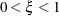, and the scale parameter _PHI_ in the GLIMMIX procedure is bounded only from below (by 0), a reparameterization is applied so that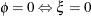andapproaches 1 asincreases. The statements preceding the calculation of the actual log likelihood are intended to prevent floating-point exceptions and to trap missing values.

Output 41.14.3 displays information about the model and estimation process. The Model Information table shows that the distribution is not a built-in distribution and echoes the expression for the user-specified variance function. As in the case of the Poisson model, the GLIMMIX procedure determines that five quadrature points are sufficient for accurate estimation of the marginal log likelihood at the starting values. The estimation process converges after 11 iterations.

Output 41.14.3: Generalized Poisson: Model, Optimization, and Iteration Information

The GLIMMIX Procedure

Model Information
Data Set WORK.COUNTS
Response Variable y
Response Distribution User specified
Variance Function _mu_ / (1-xi)/(1-xi)
Variance Matrix Blocked By sub
Estimation Technique Maximum Likelihood
Degrees of Freedom Method Containment

Optimization Information
Optimization Technique Dual Quasi-Newton
Parameters in Optimization 4
Lower Boundaries 2
Upper Boundaries 0
Fixed Effects Not Profiled
Starting From GLM estimates

Iteration History
Iteration Restarts Evaluations Objective
Function
Change Max
0 0 4 716.12976769 . 161.1184
1 0 5 716.07585953 0.05390816 11.88788
2 0 4 714.27148068 1.80437884 36.09657
3 0 2 711.02643265 3.24504804 108.4615
4 0 2 710.26952196 0.75691069 216.9822
5 0 2 709.96824991 0.30127205 96.2775
6 0 3 709.8419071 0.12634280 19.07487
7 0 3 709.83122731 0.01067980 0.649164
8 0 3 709.83047646 0.00075085 2.127665
9 0 3 709.83046461 0.00001185 0.383319
10 0 3 709.83046436 0.00000025 0.010279

The achieved –2 log likelihood is lower than in the Poisson model (compare Fit Statistics tables in Output 41.14.4 and Output 41.14.1). The scaled Pearson statistic is now less than 1.0. The fixed slope estimate remains significant at the 5% level, but the test statistics are not as large as in the Poisson model, partly because the generalized Poisson model permits more variation.

Output 41.14.4: Generalized Poisson: Fit Statistics and Estimates

Fit Statistics
-2 Log Likelihood 709.83
AIC (smaller is better) 717.83
AICC (smaller is better) 717.95
BIC (smaller is better) 723.44
CAIC (smaller is better) 727.44
HQIC (smaller is better) 719.62

Fit Statistics for Conditional Distribution
-2 log L(y | r. effects) 665.56
Pearson Chi-Square 241.42
Pearson Chi-Square / DF 0.73

Covariance Parameter Estimates
Cov Parm Subject Estimate Standard Error
Intercept sub 0.5135 0.2400
Scale   0.6401 0.09718

Solutions for Fixed Effects
Effect Estimate Standard Error DF t Value Pr > |t|
Intercept -0.7264 0.2749 29 -2.64 0.0131
x 0.003742 0.003537 299 1.06 0.2910

Based on the large difference in the –2 log likelihoods between the Poisson and generalized Poisson models, you conclude that a mixed model based on the latter provides a better fit to these data. From the Covariance Parameter Estimates table in Output 41.14.4, you can see that the estimate of the scale parameter is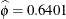and is considerably larger than 0, taking into account its standard error. The hypothesis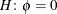, which articulates that a Poisson model fits the data as well as the generalized Poisson model, can be formally tested with a likelihood ratio test. Adding the following statement to the previous PROC GLIMMIX run compares the model to one in which the variance of the random intercepts (the first covariance parameter) is not constrained and the scale parameter is fixed at zero:

covtest 'H: phi = 0' . 0 / est;


This COVTEST statement produces Output 41.14.5.

Output 41.14.5: Likelihood Ratio Test for Poisson Assumption

Tests of Covariance Parameters
Based on the Likelihood
Label DF -2 Log Like ChiSq Pr > ChiSq Estimates H0 Note
Est1 Est2
H:phi = 0 1 854.47 144.64 <.0001 1.1959 1.11E-12 MI

MI: P-value based on a mixture of chi-squares.

Note that the –2 Log Like reported in Output 41.14.5 agrees with the value reported in the Fit Statistics table for the Poisson model (Output 41.14.2) and that the estimate of the random intercept under the null hypothesis agrees with the Covariance Parameter Estimates table in Output 41.14.2. Because the null hypothesis places the parameter(or) on the boundary of the parameter space, a mixture correction is applied in the p-value calculation. Because of the magnitude of the likelihood ratio statistic (144.64), this correction has no effect on the displayed p-value.